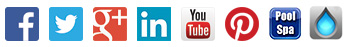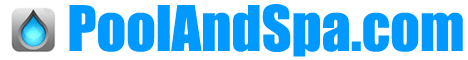. Home     How-To     Videos     Pool & Spa Products     Advanced Search     Parts     Mailing List     Order Status     Contact     View Cart .

...
Need Help?
Call 800-876-7647

 CATEGORIES Product Directory Pool & Hot Tub Parts Pool Supplies Hot Tub Supplies Hot Tub Covers Filter Cartridges Loop Loc Covers Winter Pool Covers Today's Coupons Enter Contest Pool And Spa News

 COUPONS Sign Me Up For Coupons & Special Deals From Our PoolAndSpa.com Email Newsletter Gimme My Coupons: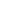# How Many Gallons Of Water Are In My Swimming Pool?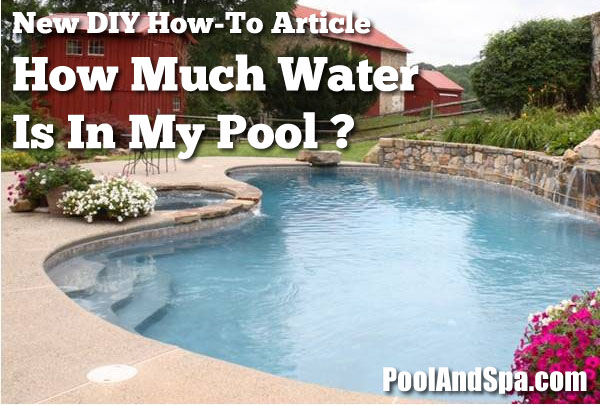How Much Water Is In My Pool? Why Do I Need To Know This, And How Do I Figure It Out? In order to figure out the proper doses of many chemicals for your pool, you often need to determine approximately how many gallons of water it holds. Also when adding a Pool Heater, Heat Pump or Solar Heater to your pool, you will also need to know how many gallons you are dealing with. To do that, you need to use a ruler to measure your pool to find out these four different numbers; Length, Width, Diameter (for Round Pools), Average Depth and then use a Fixed Volume Multiplier to determine the approximate number of gallons the pool holds. If you have never done this before, don't worry. We will walk you through the detailed steps below.

I Know What I'm Doing. Just Tell Me The Formulas.

If you don't need a detailed tutorial, and you already know your measurements, and how to calculate average depth, here are the quick formulas for you to use on your own calculator.

Formula For Average Gallons In Rectangle, Square Or Freeform Pools:

Length x Width x Average Depth x 7.5 = Total gallonsFormula For Average Gallons In Circular Pools:

Diameter x Diameter x Average Depth x 5.9 = Total gallonsFormula For Average Gallons In Oval Pools:

Length x Width x Average Depth x 5.9 = Total gallonsFormula For Average Depth Of Pools With Slopes:

Shallow End Depth + Deep End Depth Divided by 2 = Average Depth

Well, I Have The Basic Measurements, So Can You Just Figure It Out For Me?

Absolutely. You can use the E-Z Pool Water Calculator below to get the approximate number of gallons in your pool.

Tips:

1- Length And Width are for rectangle, squares or ovals. It is best to use whole numbers, rounded up. For example, if the pool is 16 1/2 feet wide, use 17 feet as the width.

2- Put the Diameter in the Length And Width fields for Circular pools. It is best to use whole numbers, rounded up. For example, if the pool is 21 1/2 feet in diameter, use 22 feet as the diameter.

3- For the Average Depth, it is best to use whole numbers, rounded up. For example, if the Average Depth is 5 1/2 feet, use 6 feet as the Average Depth.

3- Use the Gallon Multiplier of 8 for rectangles or squares for best results.

4- Use the Gallon Multiplier of 6 for Circular or Ovals for best results.

 E-Z Pool Water Calculator For The Approximate Number Of Gallons Of Water In Your Swimming Pool

One More Time - - - -  Quick Tips To Get A General Idea Using The Calculator Below:

Average Depth of a "typical" inground pool is 5
Average Depth of a "typical" above ground pool is 4
Gallon Multiplier for rectangle or square pools is 8
Gallon Multiplier for circular or oval pools is 6

Examples:

For a 16x32 rectangle inground pool you would use 16 x 32 x 5 x 8 = 20,480 Gallons
For a 24 round above ground pool you would use 24 x 24 x 4 x 6 = 13,824 Gallons

Give It A Try For Yourself !

Length = Width = X  Average Depth  =   X Gallon Multiplier =

The Approximate Gallons Of Water In Your Pool Is =

I Know Nothing. Please Walk Me Through The Whole Process.

If you know nothing, don't worry! Most people have never done this before. So we will show you the easiest and best way to measure your Pool.

Remember, No One Expects You To Be 100% Exact. Just Do The Best You Can.

Please realize that you are never going to be 100% accurate due to calculated pool water gallons (volume) are an approximate value due to the variations in the sizes, distances and angles of the various pool shapes and slopes.

Also a pool can be filled "to the top" and can also only be filled only "X" amount of inches from the top. For example, a huge rain storm can raise the water level in a pool by a few inches over night, as many people have seen all too often. And a few inches (up or down) will change the "exact" number of gallons in any pool potentially hundreds or thousands of gallons, depending on the overall size of the pool.

So don't worry. And don't get too hung up on being overly exact, just do the best you can with your measurements.What Do I Need To Measure, And How?

You can easily measure the Length, Width and Diameter (if Round) of any swimming pool with a typical ruler. Write down those measurement, generally to the nearest foot will be good enough.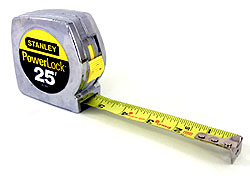Now You're Gonna Need To Get The Average Depth of the Pool

If you are lucky enough to have an Above Ground Pool, or some Inground Pools where the entire bottom is one Depth, it is very easy to figure out the Average Depth. It is just the Depth of the water. Measure it and write down that number.

However, most Inground Pools, and many newer Above Ground Pools have varying Depths, usually with angled Slopes going from level to level. This makes getting an Average Depth a bit more difficult.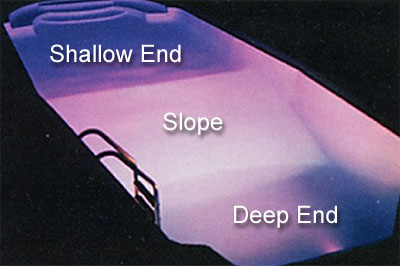How to determine the Average Depth of a Swimming Pool where the bottom slopes:

Below is a side-shot of the "typical" inground pool that has a shallow end (see "D"), then a slope that leads into the deep end (deep end is "C").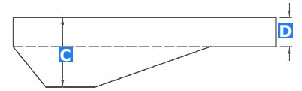If you have a long firm ruler, use that to take your measurements for the Depths. If you have a ruler, but it isn't very firm, or long enough, just use your vacuum pole to mark the depths of the pool with a simple piece of tape (or magic marker) on the pole. Then just take your measurements off the vacuum poll with your ruler. Again, you just really need the gist, don't get too hung up on an inch or two either way. Usually measurements like 3', 4', 6, 8', etc. are good enough.

First measure the depth of "D", the shallow end (usually anywhere from 3' to 5'), and then measure the depth of "C", the deep end (usually anywhere between 6' and 12'). Then, add them together and divide by 2. This is the Average Depth of your pool.

For example, if your shallow end "D" is 4', and your deep end "C" is 8', this would be your formula for the Average Depth:

Formula For Average Depth Of Pools With Slopes:

Shallow End Depth + Deep End Depth Divided by 2 = Average Depth

Example:

Shallow End = 4' + Deep End = 8', For A Total Of = 12'

12' divided by 2 = 6'

Therefore, in this example, your Average Depth would be 6 feet.

So here are the actual formulas to figure out how much water is in your swimming pool

These formulas hold true for both Inground pools as well as above ground pools.

Rectangular, Square or Free Form Pools: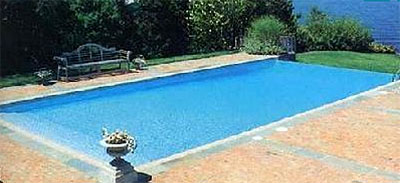Formula For Average Gallons In Rectangle, Square Or Freeform Pools:

Length x Width x Average Depth x 7.5 = Total gallons

For example: 16' x 32' x 4.5' x 7.5 = 17,280 Gallons

Circular Pools: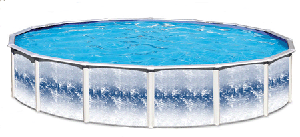Formula For Average Gallons In Circular Pools:

Diameter x Diameter x Average Depth x 5.9 = Total gallons

For example: 18' x 18' x 4' x 5.9 = 7,646 Gallons

Oval Pools: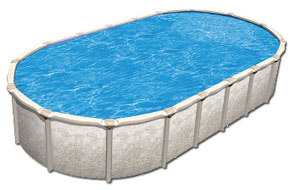Formula For Average Gallons In Oval Pools:

Length x Width x Average Depth x 5.9 = Total gallons

For example: 15' x 30' x 4' x 5.9 = 10,620 Gallons

So Now, Scroll Back Up On This Page To The E-Z Pool Water Calculator And Give It A Try!

Just take your newly discovered measurements for Length, Width, (Diameter if Round), and Average Depth - and plug those numbers into the E-Z Pool Water Calculator and see how much water is in your pool. It's that easy!

Blah, Blah, Blah. I Really Don't Have Time For All Of This. Can You Just Cut To The Chase?

If you just don't care that much about knowing the "exact" amount of water in your pool, you are in luck! We have created some handy charts of the most popular sized Pools, and their Average Gallons of water. These charts are generally accurate enough for most residential uses.

Most Common Sizes Of Inground Rectangular Pools And How Many Average Gallons They Contain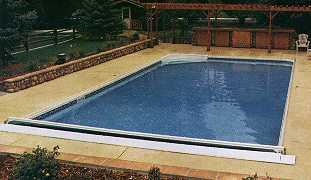POOL SIZE 4' AVG. DEPTH 5' AVG. DEPTH 6' AVG. DEPTH 12' X 24' 8,640 10,800 12,960 14' X 28' 11,760 14,700 17,640 15' X 30' 13,500 19,900 20,250 16' X 32' 15,400 19,200 23,040 18' X 36' 19,400 24,300 29,160 20' X 40' 24,000 30,000 36,000 25' X 45' 33,750 42,187 50,625 25' X 50' 37,500 46,900 56,250 30' X 50' 45,000 56,250 67,500Most Common Sizes Of Above Ground Pools And How Many Average Gallons They Contain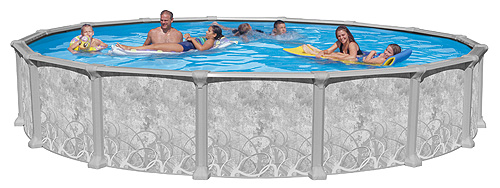Above Ground Pools with a Depth of 48" (4')

 SIZE GALLONS OF WATER 12' Round 3,398 15' Round 5,310 18' Round 7,646 21' Round 10,407 24' Round 13,593 27' Round 17,204 28' Round 18,502 30' Round 21,240 33' Round 25,700 15' x 30' Oval 10,620

Above Ground Pools with a Depth of 52" (4.5')

 SIZE GALLONS OF WATER 12' Round 3,823 15' Round 5,973 18' Round 8,602 21' Round 11,708 24' Round 15,292 27' Round 19,354 28' Round 20,815 30' Round 23,895 33' Round 28,912 15' x 30' Oval 11,947Need More Help?Can't find what you need? Feel free to give us a call.  PoolAndSpa.com's Customer Service Hotline is 1-800-876-7647 or 1-702-437-4343.